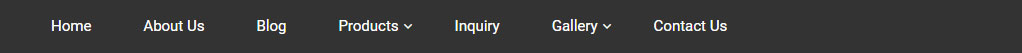Blog DetailAmfi-Registered MutualFund Distributors# Page Title### Want to know your SIP returns? Calculate it using

September 1,2021

## Want to know your SIP returns? Calculate it in 3 Simple steps using XIRR

Do you invest in mutual funds through a Systematic Investment Plan (SIP)? Or, do you invest in mutual funds from time to time? For most of us, investments and redemptions take place over a period. In that case, what is the best way to calculate the returns on your mutual fund investments?

There are different ways to calculate mutual fund returns. Compounded Annual Growth Rate (CAGR) is mostly used to calculate the returns. It is a simple formula where you take the invested amount and the current investment value into account. The formula helps to calculate point-to-point returns. However, to calculate regular or irregular investments or redemption at different points in time, you need to adopt a different method.

Extended Internal Rate of Return (XIRR) may be a better way to calculate returns on your periodic investments.

### What is XIRR?

While investing in mutual funds through SIP, you are investing regularly at a pre-determined interval. As each investment stays invested for different periods, the returns generated on these investments would differ. This is because each investment would remain invested for a different time frame. Also, the returns generated during the period would vary. Hence, to make it easier for for the purpose of calculation, we can assign an average CAGR.

We can call this adjusted CAGR as XIRR.

MS Excel automatically calculates the XIRR for you through the XIRR function.

### How is XIRR calculated?

To calculate XIRR, you need the SIP amount, date of investment, date of redemption, amount of partial redemption (if any) and the total redemption amount.

The formula for XIRR is

XIRR= XIRR (values, dates, guess)

Values are the SIP or transaction amounts, dates are the transaction dates, and guess is the approximate return you expect from the investment. You may skip the guess part.

When calculating in excel, we consider the SIPs and other investment amounts as outflow. Hence, we put a minus sign before the invested amount. Please note that there is no negative symbol for inflow or the redemption amount.

Also, make sure that you input the investment or redemption date in the dd-mm-year format as the formula may not work in other formats.

Let us take an example:

Let us assume that person A invested Rs.10,000 per month in a scheme for a year. At the beginning of the 13th month, the person redeemed the total investment worth Rs. 1.30 lakhs.

Here are the steps that you need to follow:

Step 1: Make a table with two columns. Input the date of investment/redemptions on one column and the SIP amount in the second column.

Step 2:Use the XIRR function in Excel. Now, select the values and dates. Select the range of transaction values and investment dates from the specific columns.

 Investment Date SIP Amount 10-01-2020 -10,000 10-02-2020 -10,000 10-03-2020 -10,000 10-04-2020 -10,000 10-05-2020 -10,000 10-06-2020 -10,000 10-07-2020 -10,000 10-08-2020 -10,000 10-09-2020 -10,000 10-10-2020 -10,000 10-11-2020 -10,000 10-12-2020 -10,000 10-01-2021 1,30,000 XIRR 15.66%

Step 3:Convert the value into a percentage for XIRR in percentage terms.

Here, the XIRR is 15.66%.

You can use the XIRR formula to calculate monthly SIP, yearly SIP, and returns from uneven investment amounts. Moreover, the date of investment/ redemption can also vary.

### Conclusion:

If you are a mutual fund investor, you need to know that SIP returns are not the same as the regular CAGR. XIRR is a useful MS Excel function through which you can calculate the rate of returns of your SIP instalments.

This blog is purely for educational purposes and not to be treated as personal advice. Mutual fund investments are subject to market risks, read all scheme-related documents carefully.

Tags :

All Blog